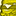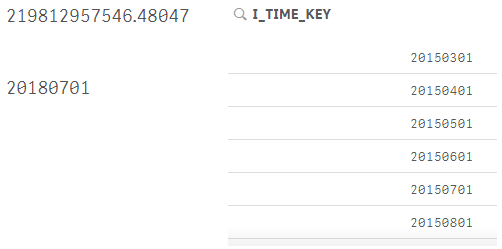# New to Qlik Sense

If you’re new to Qlik Sense, start with this Discussion Board and get up-to-speed quickly.

Announcements
QlikWorld 2023, a live, in-person thrill ride. Save \$300 before February 6: REGISTER NOW!
cancel
Showing results for
Did you mean:Contributor III

## Set analysis Query

Hi Guys,

One concern regarding set analysis need your help.

Now we set variable

vMTD

=(vCurrentYear&

(IF(vCurrentMonth='January',01,

IF(vCurrentMonth='February',02,

IF(vCurrentMonth='March',03,

IF(vCurrentMonth='April',04,

IF(vCurrentMonth='May',05,

IF(vCurrentMonth='June',06,

IF(vCurrentMonth='July',07,

IF(vCurrentMonth='August',08,

IF(vCurrentMonth='September',09,

IF(vCurrentMonth='October',10,

IF(vCurrentMonth='November',11,

IF(vCurrentMonth='December',12)))))))))))))

&01)

vCurrentYear=Year(today())

vCurrentMonth=MONTH(TODAY());

Expresion:

Sum({<[I_TIME_KEY]={"=\$(vMTD)"},V_ALIGN_FLAG={'Y'}>}N_GROSS_SALES)

you can see in the screenshot,vMTD variable works fine for 20180701, but the expresion not works, the num is the total num for

Sum({<V_ALIGN_FLAG={'Y'}>}N_GROSS_SALES), so that means set analysis [I_TIME_KEY]={"=\$(vMTD)"} not works.

Any ideas on that? Thanks.2 RepliesMVP

Two things

1) Is I_TIME_KEY a date field or number field? meaning is 20150801 is read as a number 20,150,801 or is it 01/08/2015?

2) Try one of these

Sum({<[I_TIME_KEY] = {"\$(=vMTD)"}, V_ALIGN_FLAG = {'Y'}>} N_GROSS_SALES)

or

Sum({<[I_TIME_KEY] = {"\$(=\$(vMTD))"}, V_ALIGN_FLAG = {'Y'}>} N_GROSS_SALES)Contributor III
Author

Thanks Man.

I think Sum({<[I_TIME_KEY] = {"\$(=\$(vMTD))"}, V_ALIGN_FLAG = {'Y'}>} N_GROSS_SALES) works fine, i will check.

May i know why ?

For I_Time_Key, we write date(Date#(I_Time_Key,'DD/MM/YYYY'),'YYYYMMDD') as I_Time_Key. Thanks in advance.Tags
Community Browser